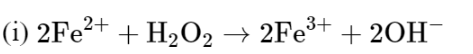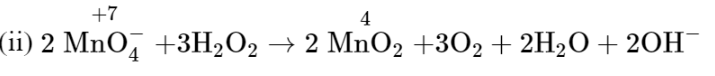# Given below are two statements:Question:

Given below are two statements:

Statement $\mathrm{I}: \mathrm{H}_{2} \mathrm{O}_{2}$ can act as both oxidising and reducing agent in basic medium.

Statement II : In the hydrogen economy, the energy is transmitted in the form of dihydrogen. In the light of the above statements, choose the correct answer from the options given below:

1. Both statement I and statement II are false

2. Both statement I and statement II are true

3.  Statement I is true but statement II is false

4. Statement I is false but statement II is true

Correct Option: , 2

Solution:

(a) $\mathrm{H}_{2} \mathrm{O}_{2}$ can acts as both oxidising and reducing agent in basic medium.In this reaction, $\mathrm{H}_{2} \mathrm{O}_{2}$ acts as oxiding agent.In this reaction, $\mathrm{H}_{2} \mathrm{O}_{2}$ acts as reducing agent.

(b) The basic principle of hydrogen economy is the transportation and

storage of energy in the form of liquids or gaseous dihydrogen. Advantage of hydrogen economy is that energy is transmitted in the form of dihydrogen and not as electric power